Introduction to Hydrology (Geog 3511)

Fall 2012

Assignment 7: GROUNDWATER

 Section Lab Assigned Due 012, 013 Tuesday Tuesday, Oct 23 Friday, Oct 26 011 Thursday Thursday, Oct 25 Monday, Oct 29

40 possible points.

CITE SOURCES OF EQUATIONS USED.

WRITE THE QUESTION AT THE START OF EACH ANSWER.

### Hydraulic Conductivity (10 Points)

Q1) Calculate the vertical flow rate (Q) using Darcy's Law for the following sandstone block. (3 points)

A equals 1.5 m x 1.5 m

the change in elevation is (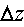) is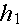is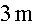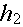is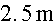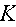is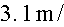day

Q2) Calculate the flow rate (Q) using Darcy's Law for a silt block. All parameters are the same except thatfor silt is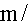day. (3 points)

Q3) Over a 24-hr period, how much more water moves through the sandstone block compared to the silt block? (2 points)

Q4) Which of these formations would be considered an aquifer and which an aquiclude? Why? (2 points)

### Unconfined Aquifer (9 points)

Given the following unconfined aquifer system:

The average width (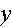) iskm

The length (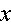) is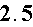km

The average thickness () is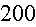m

Hydraulic conductivity () is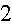m/day

The elevation at point 1 (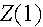) is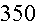m

The elevation at point 2 (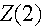) is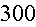m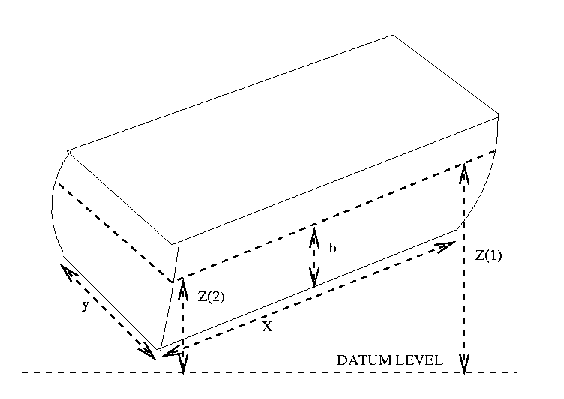Q5) Calculate the horizontal flow rate (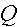) using Darcy's Law. The following image is a schematic of an unconfined aquifer from the San Luis Valley in Colorado. Which way is the water flowing and why?

Be sure to show all formulas and the calculations for each parameter in the formulas. (3 points)

Q6) What is the transmissivity of the aquifer in Q5? (2 points)

Q7) Two piezometers are installed in the unconfined aquifer. Calculate the hydraulic gradient using the data from piezometers A and B. Which way is the water flowing? Usefrom Q5. How would you use this information to calculate specific discharge? (4 points)

Piezometer A, land surface is 450 m above the datum, depth to water is 75 m.

Piezometer B, land surface is 450 m above the datum, depth to water is 150 m.

The distance between A and B is 300 m.

### Equipotential Lines, Flow Lines, and Flow Nets (10 points)

Following is an image of a plan view of a field of piezometers. Hydraulic heads at each of the wells are as follows:

A = 375 m

B = 300 m

C = 375 m

D = 400 m

E = 375 m

F = 300 m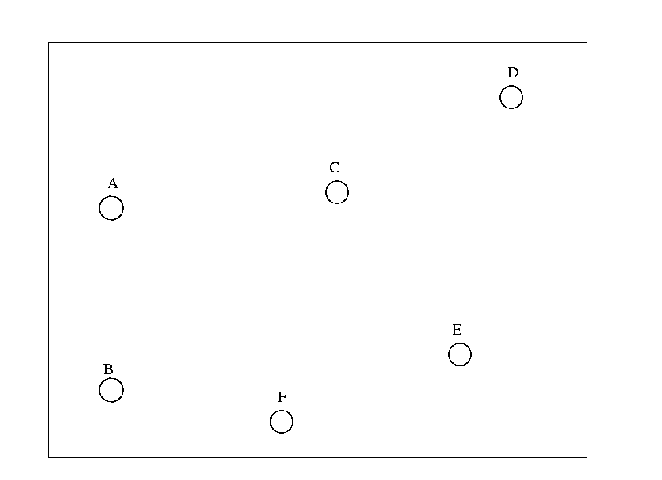Print out the image.

Q8) Add the values for hydraulic head to each of the wells

Q9) Draw equipotential lines at 300 m, 375 m, and 400 m. Use solid lines.

Q10) Draw three flowlines perpendicular to the equipotential lines, using dashed lines. One line should be to the left of C, one line should be between C and E, and one line should be to the right of the E.

Q11) On each flow, add an arrow indicating direction of flow.

### Real Case: Martinelli Snowpatch (11 points)

Lets apply our new understanding of groundwater flow to investigate a general assumption that groundwater losses from a catchment are negligible. We'll use some data from Nel Caine's research on the Martinelli catchment on Niwot Ridge. The Martinelli catchment is a small (8-ha) alpine basin with colluvium deposits at the basin outlet where the gaging station is located.

Data available:

a) Two wells located 10 m apart, in a line perpendicular to the stream.

b) The water table is 5 m lower at the well closest to the stream than at the well further away from the stream.

c) The saturated thickness of the colluvium deposit is 3 m.

d) The length (along the stream) of the colluvium deposit is 20 m.

e) Saturated hydraulic conductivity is 0.15 m/day.

f) Stream discharge () was 25 mon 21 August 1986.

Q12) What is the hydraulic gradient in the horizontal direction? (2 points)

Q13) What is the transmissivity? (2 points)

Q14) What is the volume of groundwater discharged for 21 August 1986 in cubic meters? (3 points)

Q15) What is the ratio of groundwater discharge to stream discharge for that day? (2 points)

Q16) In the simplified hydraulic equation (drats, vertical learning),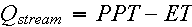and the groundwater component (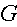or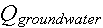) is ignored. Is that assumption valid here? Why or why not? (2 points)